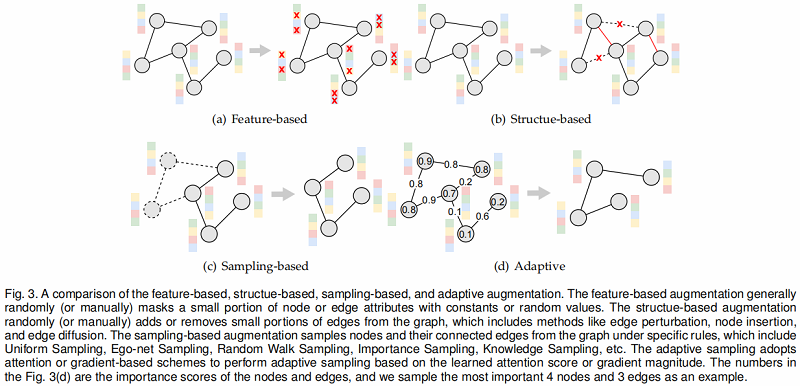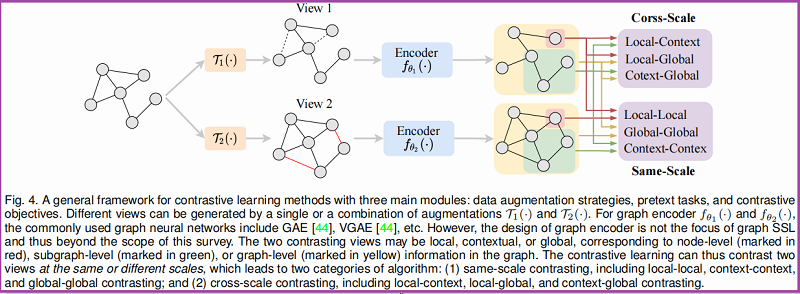# 3 对比学习

## 3.1 统一的视角

对比学习的主要目标是最大化正对之间的一致性，负对的非一致性。

对比学习步骤总结如下：

首先，给定一张图 $g=(\mathbf{A}, \mathbf{X})$, $K$ 个不同的变换 $\mathcal{T}_{1}, \mathcal{T}_{1}, \cdots, \mathcal{T}_{K}$ 被用于产生产生多个视图 $\left\{\left(\mathbf{A}_{k}, \mathbf{X}_{k}\right)\right\}_{k=1}^{K}$，定义如下：

$\mathbf{A}_{k}, \mathbf{X}_{k}=\mathcal{T}_{k}(\mathbf{A}, \mathbf{X}) ; k=1,2, \cdots, K$

其次，对每一个视图，一系列的 graph Encoder $\left\{f_{\theta_{k}}\right\}_{k=1}^{K}$（可能共享权值也可能一样） 被用于产生不同的表示 $\mathbf{h}_{1}, \mathbf{h}_{2}, \cdots, \mathbf{h}_{K}$ ，即

$\mathbf{h}_{k}=f_{\theta_{k}}\left(\mathbf{A}_{i}, \mathbf{X}_{i}\right) ; k=1,2, \cdots, K$

最后，对比学习的目的是最大化来自同一实例的两个视图的互信息：

$\underset{\theta_{1}, \theta_{2}, \cdots, \theta_{K}}{\text{max}} \sum\limits_{i} \sum\limits _{j \neq i} \alpha_{i, j} \mathcal{M I}\left(\mathbf{h}_{i}, \mathbf{h}_{j}\right)$

其中，$i, j \in\{1,2, \cdots, K\}$，$\left\{\mathbf{h}_{i}\right\}_{i=1}^{K}$  从 $g=(\mathbf{A}, \mathbf{X})$ 产生 , 且被认为是正样本。$\mathcal{M I}\left(\mathbf{h}_{i}, \mathbf{h}_{j}\right)$ 表示 $\mathbf{h}_{i}$  和  $\mathbf{h}_{j}$ 之间的互信息。对于不同的代理任务，$\left\{\mathbf{h}_{k}\right\}_{k=1}^{K}$ 可能不是一个尺度的，可以作为节点级、子图级或图级的表示。与正样本 $\left\{\mathbf{h}_{i}\right\}_{i=1}^{K}$ 对立的负样本 $\left\{\widetilde{\mathbf{h}}_{i}\right\}_{i=1}^{K}$ 是从其他图 $\widetilde{g}=(\widetilde{\mathbf{A}}, \widetilde{\mathbf{X}})$ 制造出来的。这里的 $\alpha_{i, j} \in\{0,1\}$ 根据不同的方案进行设计。

图数据对比学习的设计可概括为三个主要模块：（1）数据增强策略、（2）代理和（3）对比目标。

## 3.2 数据增强

在这里，我们将图数据的数据增强策略分为四类：
• feature-based
• structure-based
• sampling-based
Fig. 3 显示了四种增强类型的概述。### 3.2.1 Feature-based Augmentation

给定一个输入图$(\mathbf{A}, \mathbf{X})$，一个基于特征的增强只对节点特征矩阵 $X$ 或边特征矩阵 $\mathbf{X}^{e}$ 进行变换。不失一般性，我们以$\mathbf{X}$ 为例，由

$\widetilde{\mathbf{A}}, \tilde{\mathbf{X}}=\mathcal{T}(\mathbf{A}, \mathbf{X})=\mathbf{A}, \mathcal{T}_{\mathbf{X}}(\mathbf{X})\quad\quad\quad(12)$

Attribute Masking 随机掩蔽了一小部分属性。我们将 Attribute Masking $\mathcal{T}_{\mathbf{X}}(\mathbf{X})$ 定义为：

$\mathcal{T}_{\mathbf{X}}(\mathbf{X})=\mathbf{X} \odot(1-\mathbf{L})+\mathbf{M} \odot \mathbf{L}\quad\quad\quad(13)$

其中

$\mathbf{L}$ 是掩蔽位置矩阵（masking location matrix），当 $v_{i}$ 的第 $j$ 个元素被隐藏的时候 $\mathbf{L}_{i, j}=1$，否则 $\mathbf{L}_{i, j}=0$

其中

• $\mathbf{L}$ 是掩蔽位置矩阵（masking location matrix），当 $v_{i}$ 的第 $j$ 个元素被隐藏的时候 $\mathbf{L}_{i, j}=1$，否则 $\mathbf{L}_{i, j}=0$；
• $\mathbf{M}$ 是掩蔽值矩阵（masking value matrix）；

矩阵 $\mathbf{L}$ 通常通过伯努利分布生成或者自定义设置，比如

• $\mathbf{M}=\mathbf{0}$ 表示恒定掩蔽；
• $\mathbf{M} \sim N(\mathbf{0}, \boldsymbol{\Sigma})$ 将原始输入替换为带高斯噪声；
• $\mathbf{M} \sim N(\mathbf{X}, \boldsymbol{\Sigma})$ 代表在输入上添加高斯噪声；

3.2.1.2 Attribute Shuffling

Attribute Shuffling 对属性矩阵 $X$ 进行行变换，也就是说，增广图由与原始图相同的节点组成，但它们位于图的不同位置，接收不同的上下文信息。

$\mathcal{T}_{\mathbf{X}}(\mathbf{X})$ 被定义为

$\mathcal{T}_{\mathbf{X}}(\mathbf{X})=\mathbf{X}[i d x,:]\quad\quad\quad(14)$

其中 $\text{idx}$ 是一个包含 $1-N$ 的列表，但是经过随机打乱

### 3.2.2 Structue-based Augmentation

给定一个图 $(\mathbf{A}，\mathbf{X})$，一个基于结构的增强器只对相邻的矩阵 $\mathbf{A}$ 进行转换，如下所示

$\tilde{\mathbf{A}}, \tilde{\mathbf{X}}=\mathcal{T}(\mathbf{A}, \mathbf{X})=\mathcal{T}_{\mathbf{A}}(\mathbf{A}), \mathbf{X}\quad\quad\quad(15)$

3.2.2.1 Edge Perturbation

通过随机添加或去除一定比例的边来扰动结构上的连通性。我们指定边扰动的 $\mathcal{T}_{\mathbf{A}}(\mathbf{A})$ 为

$\mathcal{T}_{\mathbf{A}}(\mathbf{A})=\mathbf{A} \odot(1-\mathbf{L})+(1-\mathbf{A}) \odot \mathbf{L}\quad\quad\quad(16)$

其中 $\mathbf{L}$ 是一个扰动位置矩阵，当 $v_{i}$ 和 $v_{j}$ 之间的边被扰动的时候 $\mathbf{L}_{i, j}= \mathbf{L}_{j, i}=1$，否则 $\mathbf{L}_{i, j}=\mathbf{L}_{j, i}=0$；

3.2.2.2 Node Insertion

节点插入，添加 $K$ 个节点 $\mathcal{V}_{a}=\left\{v_{N+k}\right\}_{k=1}^{K}$ 到节点集 $\mathcal{V}$ 且在$\mathcal{V}_{a}$ 和 $\mathcal{V}$ 之间添加一些边。对于结构转换 $\widetilde{\mathbf{A}}=\mathcal{T}_{\mathbf{A}}(\mathbf{A})$，我们有 $\widetilde{\mathbf{A}}_{: N,: N}=\mathbf{A}$.。给定连接比率$r$，我们有

$p\left(\widetilde{\mathbf{A}}_{i, j}=\widetilde{\mathbf{A}}_{j, i}=1\right)=r, p\left(\widetilde{\mathbf{A}}_{i, j}=\widetilde{\mathbf{A}}_{j, i}=0\right)=1-r \quad\quad\quad(17)$
其中：$N+1 \leq i, j \leq N+K$

3.2.2.3 Edge Diffusion

边缘扩散生成原始图结构的不同拓扑视图，一般的边缘扩散过程定义为

$\mathcal{T}_{\mathbf{A}}(\mathbf{A})=\sum_{k=0}^{\infty} \Theta_{k} \mathbf{S}^{k}\quad\quad\quad(18)$

其中：
$\mathbf{S} \in \mathbb{R}^{N \times N}$ 是广泛的转移矩阵，常用的两种(1) Personalized PageRank (PPR)；(2) Heat Kernel (HK)；
$\Theta$ 是加权系数，且$\sum_{k=0}^{\infty} \Theta_{k}= 1$，$\Theta_{k} \in[0,1]$ ；

对于PPR：

$\mathcal{T}_{\mathbf{A}}^{P P R}(\mathbf{A}) =\alpha\left(\mathbf{I}_{n}-(1-\alpha) \mathbf{D}^{-1 / 2} \mathbf{A} \mathbf{D}^{-1 / 2}\right)^{-1}$
且 $\mathbf{S}=\mathbf{D}^{-1 / 2} \mathbf{A D}^{-1 / 2}$，$\Theta_{k}=\alpha(1-\alpha)^{k}$

对于 HK：

$\mathcal{T}_{\mathbf{A}}^{H K}(\mathbf{A}) =\exp \left(t \mathbf{A} \mathbf{D}^{-1}-t\right)$
且 $\mathbf{S}=\mathbf{A D}^{-1}$，$\Theta_{k}=e^{-t} t^{k} / k !$

### 3.2.3 Sampling-based Augmentation

给定一个输入图 $(A，X)$，一个基于采样的增强器对相邻的矩阵 $A$ 和特征矩阵 $X$ 都进行变换，如下所示

$\widetilde{\mathbf{A}}, \tilde{\mathbf{X}}=\mathcal{T}(\mathbf{A}, \mathbf{X})=\mathbf{A}[\mathcal{S}, \mathcal{S}], \mathbf{X}[\mathcal{S},:]\quad\quad\quad(20)$

其中：$\mathcal{S} \in \mathcal{V}$。

这里列出 $5$ 种常用的采样策略去获得$\mathcal{S}$：

• uniform sampling
• ego-nets sampling
• random walk sampling
• importance sampling
• knowledge-based sampling

3.2.3.1 Uniform Sampling

均匀采样从 $\mathcal{V}$ 中均匀采样给定数量的节点 $S$，并直接去除剩余的节点。

3.2.3.2 Ego-nets Sampling

给定一个典型的具有 $L$ 层的图编码器，节点表示的计算只依赖于它的 $\text{L-hop}$ 邻域。特别的对于每个节点$v_i$，转移矩阵$\mathcal{T}(\cdot)$采样 $v_{i}$ 的 $\text{L-hop}$ 邻居。这里 $\mathcal{S}$ 定义为：

$\mathcal{S}=\left\{v_{j} \mid d\left(v_{i}, v_{j}\right) \leq L\right\}\quad\quad\quad(21)$

其中，$d\left(v_{i}, v_{j}\right)$ 是节点 $v_{i}$ 和 $v_{j}$ 之间的最短路径。

Ego-nets Sampling本质上是广度优先搜索(BFS)抽样的一个特殊形式。

3.2.3.3 Random Walk Sampling

从节点$v_i$ 开始在图 $g$ 上进行随机游走。该行走以与边权值成正比的概率迭代地移动到它的邻域。此外，在每一步中，行走以一个正的概率 $\alpha$ 返回到起始节点 $v_i$。最后，将被访问的节点收集到一个节点子集 $S$ 中。

3.2.3.4 Importance Sampling

给定一个节点 $v_i$，我们可以根据相邻节点的重要性对子图进行采样，重要得分矩阵 $M$ 定义为

$\mathbf{M}=\alpha \cdot\left(\mathbf{I}_{n}-(1-\alpha) \cdot \mathbf{A} \mathbf{D}^{-1}\right)\quad\quad\quad(22)$

其中，$\alpha \in[0,1]$

对于给定的节点 $v_{i}$，子图采样器选择由$v_{i}$ 锚定的 $top- k$ 个重要邻居组成一个子图，所选节点的索引表示为 $\mathcal{S}= \text{top_rank} (\mathbf{M}(i,:), k)$。

3.2.3.5 Knowledge Sampling

基于知识的抽样将领域知识整合到子图抽样中。例如，采样过程可以通过计算分子图中经常发生的子结构和生物信息学子结构，从而形式化为基于库的匹配问题。

### 3.2.4 Adaptive Augmentation

通常使用注意力分数或梯度来指导节点或边的选择。

3.2.4.1 Attention-based

基于注意力的方法通常为节点或边定义重要性分数，然后根据它们的重要性来数据增强。

例如，GCA建议保留重要的结构和属性不变，同时干扰可能不重要的边缘和特征。具体来说，边去除和特征掩蔽的概率应该与它们的重要性密切相关。给定一个节点中心性度量 $\varphi_{c}(\cdot): \mathcal{V} \rightarrow \mathbb{R}^{+}$ ，它将边中心性定义为两个相邻节点的中心性得分的平均值$s_{i, j}=\log \frac{\varphi_{c}\left(v_{i}\right)+\varphi_{c}\left(v_{j}\right)}{2}$，边 $e_{i, j}$ 的重要性定义为：

$p_{i, j}=\min \left(\frac{s_{\max }-s_{i, j}}{s_{\max }-\mu_{s}} \cdot p_{e}, p_{\tau}\right)\quad\quad\quad(23)$

其中，$p_{e}$ 是一个控制去除边的总体概率的超参数，$s_{\max }$ 和 $\mu_{s}$ 是 $\left\{s_{i, j}\right\}_{j=1}^{N}$ 的最大值和平均值。$p_{\tau}<1$ 是一个截止概率，用于截断概率，因为极高的去除概率会过度破坏图的结构。

节点中心性可以定义为度中心性、特征向量中心性或 PageRank 中心性，从而产生三个变量。基于节点重要性的属性屏蔽与上面相同，并且不会被重复。

与 GRACE 中简单的均匀边去除和插入不同，GROC在边梯度信息的引导下自适应地执行基于梯度的增强。具体来说，它首先将两个随机变换 $\mathcal{T}_{1}(\cdot)$ 和$\mathcal{T}_{2}(\cdot)$ 应用于图 $g= (\mathbf{A}, \mathbf{X})$，得到两个视图，以概率 $r_{1}$和 $r_{2}$ 独立屏蔽节点属性，然后计算这两个视图之间的对比损失 $\mathcal{L}_{s s l}$。对于给定的节点 $v_{i}$，将一个边去除候选集定义为

$\mathcal{S}^{-}=\left\{\left(v_{i}, v_{k}\right) \mid v_{k} \in \mathcal{N}_{i}^{(l)}\right\}\quad\quad\quad(24)$

并将一个边插入候选集定义为

$\mathcal{S}^{+}=\left\{\left(v_{i}, v_{k}\right) \mid v_{k} \in\left(\cup_{v_{m} \in \mathcal{B}} \mathcal{N}_{m}^{(l)} \backslash \mathcal{N}_{i}^{(l)}\right)\right\}\quad\quad\quad(25)$

其中：$\mathcal{B} \subset \mathcal{V}$ 是一个包含节点的 $\text{batch}$。
$\mathcal{S}^{+}$ 被边集合 $\left(v_{i}, v_{k}\right)$ 限制，这里 $v_{i}$ 作为anchor node，$v_{k}$ 在其他一些锚点 $v_{m} \neq v_{i}$ 的 $\text{l-hop}$ 邻域内，但不在节点 $v_{i}$ 的$\text{l-hop}$ 邻域内。

最后，我们反向传播损失 $\mathcal{L}_{s s l}$，得到 $\mathcal{S}^{-}$ 和 $\mathcal{S}^{+}$ 中每条边的梯度强度值。通过在 $\mathcal{S}^{-}$ 中去除具有最小边梯度幅度值的边缘子集，并在$\mathcal{S}^{+}$ 中插入具有最大边缘梯度幅度值的边子集，在 $\mathcal{S}^{+}$ 中插入具有最大边缘梯度幅度值的边子集。

## 3.3 Pretext Task

对比学习的目的是使两个联合抽样的正对的一致性最大化。根据图视图的定义，视图的尺度可以是局部的、上下文的或全局的，分别对应于图中的节点级、子图级或图级。

因此，对比学习可以在相同或不同的尺度上对比两个图视图，从而导致两类：（1）同尺度对比，（2）不同尺度对比。

1.同尺度对比

• node-node
• graph-graph

2.不同尺度对比

• node-subgraph
• node-graph contrasting

我们从这两个角度对现有的方法进行分类，并以一个统一的框架呈现，如图4所示### 3.3.1 Contrasting with the same-scale

相同尺度的对比学习根据观点的不同尺度进一步细化为三类：local-local、context-context 和 global-global 对比。

3.3.1.1 Global-Global Contrasting

GraphCL

采用四种类型的图增强 $\left\{\mathcal{T}_{k}\right\}_{k=1}^{4}$ 来合并各种先验：(1) Node Dropping  $\mathcal{T}_{1}(\cdot)$ ; (2) Edge Perturbation  $\mathcal{T}_{2}(\cdot)$ ; (3) Attribute Masking  $\mathcal{T}_{3}(\cdot)$ ; (4) Subgraph Sampling  $\mathcal{T}_{4}(\cdot)$。

给定一个图$g_{i}=\left(\mathbf{A}_{i}, \mathbf{X}_{i}\right) \in \mathcal{G}$，它首先应用一系列图增强 $\mathcal{T}(\cdot)$，随机选择从 $\left\{\mathcal{T}_{k}\right\}_{k=1}^{4}$ 生成一个增强图$\widetilde{g}_{i}=\left(\widetilde{\mathbf{A}}_{i}, \widetilde{\mathbf{X}}_{i}\right)=\mathcal{T}\left(\mathbf{A}_{i}, \mathbf{X}_{i}\right)$，然后学习预测两个图是否来自同一图。具体来说，这是一个共享的图级 Encoder  $f_{\gamma}(\cdot)$  用于获得图级表示 $\mathbf{h}_{g_{i}}=f_{\gamma}\left(\mathbf{A}_{i}, \mathbf{X}_{i}\right)$  且   $\widetilde{\mathbf{h}}_{\tilde{g}_{i}}=f_{\gamma}\left(\widetilde{\mathbf{A}}_{i}, \widetilde{\mathbf{X}}_{i}\right)$。最后，学习目标的定义如下

$\underset{\theta}{\text{max}} \frac{1}{|\mathcal{G}|} \sum\limits _{g_{i} \in \mathcal{G}} \mathcal{M I}\left(\mathbf{h}_{g_{i}}, \widetilde{\mathbf{h}}_{\tilde{g}_{i}}\right)\quad\quad\quad(26)$

Contrastive Self-supervised Learning (CSSL)

对比自监督学习(CSSL)遵循一个与 GraphGL 非常相似的框架，不同的只是数据增强的方式。不仅有节点的删除，它还认为节点插入是一种重要的增强策略。具体来说，它随机选择一个强连通的子图 $S$，去除 $S$ 中的所有边，添加一个新的节点 $v_i$，并在 $v_i$ 和 $S$ 中的每个节点之间添加一条边。

Label Contrastive Coding (LCC)

提出了标签对比编码(LCC)，以鼓励类内的紧凑性和类间的可分离性。

为了增强对比学习，LLC 引入了一个动态标签存储库（dynamic label memory bank ）和一个动量更新编码器（momentum updated encoder）。具体来说，查询图（$\left(g_{q}, y_{q}\right)$） $\left(g_{q}, y_{q}\right)$ 和关键图（key graph）$\left(g_{k}, y_{k}\right)$由两个图级编码器 $f_{\gamma_{q}}(\cdot)$  和  $f_{\gamma_{k}}(\cdot)$ 进行编码，分别得到图级表示 $\mathbf{h}_{g_{q}}$  和  $\mathbf{h}_{g_{k}}$。如果 $\mathbf{h}_{g_{q}}$  和 $\mathbf{h}_{g_{k}}$ 具有相同的标签，则认为它们为正对，否则就认为它们为负对。标签对比损失鼓励模型来区分正对和负对。对于编码查询$\left(g_{q}, y_{q}\right)$，其标签对比损失计算为

${\large \underset{\gamma_{q}}{\text{max}}\log \frac{\sum\limits_{i=1}^{m} \mathbb{I}_{y_{i}=y_{q}} \cdot \exp \left(\mathbf{h}_{g_{q}} \cdot \mathbf{h}_{g_{k}}^{(i)} / \tau\right)}{\sum\limits _{i=1}^{m} \exp \left(\mathbf{h}_{g_{q}} \cdot \mathbf{h}_{g_{k}}^{(i)} / \tau\right)}} \quad\quad\quad(27)$

其中 $m$ 代表 $\text{memory bank}$ 的大小，$\mathbb{I}_{y_{i}=y_{q}}$ 是一个指示器函数（indicator function），用于确定内存库中第 $i$ 个关键图 $g_{k}^{(i)}$ 的标签是否与 $y_{q}$ 相同。$f_{\gamma_{k}}(\cdot)$ 的参数 $\gamma_{k}$ 遵循一个基于动量的更新机制，如 Moco 。

$\gamma_{k} \longleftarrow-\alpha \gamma_{k}+(1-\alpha) \gamma_{q}\quad\quad\quad(28)$

其中，$\alpha \in[0,1)$ 是控制 $\gamma_{k}$ 演化速度的动量权重。

3.3.1.2 Context-Context Contrasting

Graph Contrastive Coding (GCC)

图对比编码(GCC)是一个图自监督的预训练框架，它捕获了多个图的图拓扑属性。具体来说，它首先图为每个图 $g∈G$ 采样多个基于随机游走的子图，并放在一个 memory bank $S$。查询子图（query subgraph） $g_q \in S$ 和关键子图（key subgraph）$g_k \in S$ 由两个图级编码器 $f_{\gamma_{q}(\cdot)}$ 和 $f_{\gamma_{k}}(\cdot)$ 编码，并分别获得图级表示$\mathbf{h}_{g_{q}}$和 $\mathbf{h}_{g_{k}}$。如果 $g_q$ 和 $g_k$ 从同一个图中采样，则它们被认为是正对，否则它们是负对。对于编码查询 $(g_q，y_q)$，其中 $y_q$ 是它从其中采样的图的索引，它的图的对比损失被计算为

${\large \underset{\gamma_{q}}{\text{max} } \quad \log \frac{\sum\limits _{i=1}^{|\mathcal{S}|} \mathbb{I}_{y_{i}=y_{q}} \cdot \exp \left(\mathbf{h}_{g_{q}} \cdot \mathbf{h}_{g_{k}}^{(i)} / \tau\right)}{\sum\limits_{i=1}^{|\mathcal{S}|} \exp \left(\mathbf{h}_{g_{q}} \cdot \mathbf{h}_{g_{k}}^{(i)} / \tau\right)}}\quad\quad\quad(29)$

其中，$\mathbb{I}_{y_{i}=y_{q}}$ 是一个指示器函数，用于确定 memory bank 和 query graph $g_{q}$中的第 $i$ 个key graph $g_{k}^{(i)}$ 是否从同一图中采样。$f_{\gamma_{k}}(\cdot)$ 的参数 $\gamma_{k}$ 遵循基于动量的表达式形式的更新。

3.3.1.3 Local-Local Contrasting

GRACE

GRACE 侧重于节点级别上的对比。给定一个图 $g=(\mathbf{A}, \mathbf{X})$，它首先生成两个扩充图 $g^{(1)}=\left(\mathbf{A}^{(1)}, \mathbf{X}^{(1)}\right)=\mathcal{T}_{1}(\mathbf{A}, \mathbf{X})$ 和 $g^{(2)}=\left(\mathbf{A}^{(1)}, \mathbf{X}^{(2)}\right)= \mathcal{T}_{2}(\mathbf{A}, \mathbf{X})$。然后应用一个共享编码器 $f_{\theta}(\cdot)$ 生成它们的节点嵌入矩阵 $\mathbf{H}^{(1)}=f_{\theta}\left(\mathbf{A}^{(1)}, \mathbf{X}^{(1)}\right)$ 和 $\mathbf{H}^{(2)}=f_{\theta}\left(\mathbf{A}^{(2)}, \mathbf{X}^{(2)}\right)$。最后，每个正对的成对目标 $\left(\mathbf{h}_{i}^{(1)}, \mathbf{h}_{i}^{(2)}\right)$ 定义如下：

${\large \mathcal{L}\left(\mathbf{h}_{i}^{(1)}, \mathbf{h}_{i}^{(2)}\right)=\log \frac{e^{\mathcal{D}\left(\mathbf{h}_{i}^{(1)}, \mathbf{h}_{i}^{(2)}\right) / \tau}}{e^{\mathcal{D}\left(\mathbf{h}_{i}^{(1)}, \mathbf{h}_{i}^{(2)}\right) / \tau}+N e g}}\quad\quad\quad(30)$

其中，$N e g$ 的定义：

$N e g=\sum\limits _{k=1}^{N} \mathbf{1}_{k \neq i}\left[e^{\mathcal{D}\left(\mathbf{h}_{i}^{(1)}, \mathbf{h}_{k}^{(1)}\right) / \tau}+\mid e^{\mathcal{D}\left(\mathbf{h}_{i}^{(1)}, \mathbf{h}_{k}^{(2)}\right) / \tau}\right]\quad\quad \quad\quad(31)$

其中，$e^{\mathcal{D}\left(\mathbf{h}_{i}^{(1)}, \mathbf{h}_{k}^{(1)}\right) / \tau}$ 为视图内负对，$e^{\mathcal{D}\left(\mathbf{h}_{i}^{(1)}, \mathbf{h}_{k}^{(2)}\right) / \tau}$ 为视图间负对。那么，要最大化的总体目标被定义为：

$\underset{\theta}{\text{max}} \frac{1}{2 N} \sum\limits _{i=1}^{N}\left[\mathcal{L}\left(\mathbf{h}_{i}^{(1)}, \mathbf{h}_{i}^{(2)}\right)+\mathcal{L}\left(\mathbf{h}_{i}^{(2)}, \mathbf{h}_{i}^{(1)}\right)\right]\quad\quad\quad\quad(32)$

GCA 和 GROC

GCA 和 GROC 采用了与 GRACE 相同的框架和目标，但具有更灵活和自适应的数据增强策略。SEPT 提出的框架类似于GRACE，但它是专门为特定的下游任务（推荐）设计的，通过将不同视图对比学习与半监督三训练相结合。从技术上讲，SEPT 首先用用户的社会信息来增强用户数据，然后在增强的视图上构建三个图编码器，一个用于推荐，另外两个用于预测未标记的用户。给定某个用户，SEPT 将预测标签与目标用户高度一致的节点作为正样本，然后鼓励目标用户与正样本之间的一致性。

Cross-layer Contrasting (GMI)

给定一个图 $g=(\mathbf{A}, \mathbf{X})$，利用图编码器 $f_{\theta}(\cdot)$ 得到节点嵌入矩阵 $\mathbf{H}=f_{\theta}(\mathbf{A}, \mathbf{X})$。然后交叉层节点对比可以定义为

$\underset{\theta}{\text{max}} \frac{1}{N} \sum\limits _{i=1}^{N} \mathcal{M I}\left(\mathbf{h}_{i}, \mathbf{x}_{i}\right)\quad\quad\quad(33)$

其中，与 $\mathbf{h}_{i}$ 形成对比的负样本是 $\operatorname{Neg}\left(\mathbf{h}_{i}\right)= \left\{\mathbf{x}_{j} \mid v_{j} \in \mathcal{N}_{i}\right\}$。类似地，跨图层的边缘对比度可以被定义为

$\underset{\theta}{\text{max}} \frac{1}{N} \sum\limits _{i=1}^{N} \sum\limits_{v_{j} \in \mathcal{N}_{i}} \mathcal{M I}\left(\mathbf{w}_{i, j}, \mathbf{A}_{i, j}\right)\quad\quad\quad(34)$

其中，$\mathbf{w}_{i, j}=\sigma\left(\mathbf{h}_{i} \mathbf{h}_{j}^{T}\right)$ 和与 $\mathbf{w}_{i, j}$ 形成对比的负样本为 $N e g\left(\mathbf{w}_{i, j}\right)=\left\{\mathbf{A}_{i, k} \mid v_{k} \in \mathcal{N}_{i}\right. and \left.k \neq j\right\}$ 。

STDGI

将互信息最大化的思想扩展到时空图（spatial-temporal graphs）中。具体来说，给定 $t$ 和 $t+k$ 时刻的两个图 $g_{t}=\left(\mathbf{A}, \mathbf{X}^{(t)}\right)$ 和 $g_{t+k}=\left(\mathbf{A}, \mathbf{X}^{(t+k)}\right)$，利用共享图编码器 $f_{\theta}(\cdot)$ 得到节点嵌入矩阵 $\mathbf{H}^{(t)}=f_{\theta}\left(\mathbf{A}, \mathbf{X}^{(t)}\right)$。此外，它通过随机排列节点特征生成一个增强图，得到 $\widetilde{g}_{t+k}=\left(\mathbf{A}, \tilde{\mathbf{X}}^{(t+k)}\right)= \mathcal{T}\left(\mathbf{A}, \mathbf{X}^{(t+k)}\right)$。最后，将学习目标定义如下

$\underset{\theta}{\text{max}} \frac{1}{N} \sum\limits _{i=1}^{N} \mathcal{M} \mathcal{I}\left(\mathbf{h}_{i}^{(t)}, \mathbf{x}_{i}^{(t+k)}\right)\quad\quad\quad(35)$

其中，与 $\mathbf{h}_{i}^{(t)}$ 相比的负样本是 $\operatorname{Neg}\left(\mathbf{h}_{i}^{(t)}\right)=\widetilde{\mathbf{x}}_{i}^{(t+k)}$。

BGRL

受 BYOL 的启发，BGRL提出执行不需要负样本的自监督学习，从而摆脱潜在的二次瓶颈。具体来说，给定一个图 $g=(\mathbf{A}, \mathbf{X})$，它首先生成两个增强图视图 $g^{(1)}=\left(\mathbf{A}^{(1)}, \mathbf{X}^{(1)}\right)= \mathcal{T}_{1}(\mathbf{A}, \mathbf{X})$ 和 $g^{(2)}=\left(\mathbf{A}^{(1)}, \mathbf{X}^{(2)}\right)=\mathcal{T}_{2}(\mathbf{A}, \mathbf{X})$。然后应用两个图编码器 $f_{\theta_{1}}(\cdot)$ 和 $f_{\theta_{2}}(\cdot)$ 来生成它们的节点嵌入矩阵 $\mathbf{H}^{(1)}=f_{\theta_{1}}\left(\mathbf{A}^{(1)}, \mathbf{X}^{(1)}\right)$ 和 $\mathbf{H}^{(2)}=f_{\theta_{2}}\left(\mathbf{A}^{(2)}, \mathbf{X}^{(2)}\right)$ 。此外，使用节点级预测头 $g_{\omega}(\cdot)$ 输出 $\mathbf{Z}^{(1)}=g_{\omega}\left(\mathbf{H}^{(1)}\right)$ 。最后，将学习目标定义如下

${\large \underset{\theta_{1}, \omega}{\text{max}} \frac{1}{N} \sum\limits _{i=1}^{N} \frac{\mathbf{z}_{i}^{(1)}\left(\mathbf{h}_{i}^{(2)}\right)^{T}}{\left\|\mathbf{z}_{i}^{(1)}\right\|\left\|\mathbf{h}_{i}^{(2)}\right\|}}\quad\quad \quad\quad(36)$

其中，参数 $\theta_{2}$ 被更新为参数 $\theta_{1}$ 的指数移动平均数(EMA)，如在 $\text{Eq. 28}$ 中所做的那样。

SelfGNN

与 BGRL 的不同之处仅在于目标函数的定义。与 $\text{Eq. 36}$ 不同。SelfGNN 直接以 MSE 的形式定义了隐式对比项：

$\underset{\theta_{1}, \omega}{\text{min}}\frac{1}{N} \sum\limits _{i=1}^{N}\left\|\mathbf{z}_{i}^{(1)}-\mathbf{h}_{i}^{(2)}\right\|^{2}\quad\quad\quad\quad(37)$

其中，与 $\mathbf{h}_{i}$ 形成对比的负样本是 $N e g\left(\mathbf{h}_{i}\right)=\left\{\mathbf{z}_{j}\right\}_{v_{j} \in \mathcal{V}, j \neq i}$ 。

HeCo

考虑元路径集 $\Phi_{k}$ 中的元路径 $\left\{\Phi_{k}\right\}_{k=1}^{K}$ ，如果节点 $v_{i}$ 和节点 $v_{j}$ 之间存在元路径 $v_{i}$，那么 $v_{j}$ 可以看作是在节点 $v_{i}$ 的元路径邻域 $\mathcal{N}_{i}^{\Phi_{k}}$ 中，从而生成一个基于相邻矩阵的元路径 $\mathbf{A}^{\Phi_{k}}$。HeCo 首先应用两个图编码器$f_{\theta_{1}}^{s c}(\cdot)$ 和 $f_{\theta_{2}}^{m p}(\cdot)$ 获得节点嵌入$\mathbf{H}^{s c}=f_{\theta_{1}}^{s c}(\mathbf{A}, \mathbf{X})$ 和 $\mathbf{H}^{m p}=f_{\theta_{2}}^{m l}\left(\left\{\mathbf{A}^{\Phi_{k}}\right\}_{k=1}^{K}, \mathbf{X}\right)$ ，然后为定义正样本和负样本，HeCo首先定义一个函数 $\mathbb{C}_{i}(j)=\sum_{k=1}^{K} \mathbb{I}\left(j \in \mathcal{N}_{i}^{\Phi_{k}}\right)$来计算连接节点$v_{i}$ 和 $v_{j}$ 的元路径的数量。然后构造一个集合 $\mathcal{S}_{i}=\left\{j \mid j \in \mathcal{V} \text { and } \mathbb{C}_{i}(j) \neq 0\right\}$ 和 $\left.\mathbb{C}_{i}(j) \neq 0\right\}$，并根据 $\mathbb{C}_{i}(j)$ 的值对其进行降序排序。然后从 $\mathcal{S}_{i}$ 中选择顶部的 $T_{p o s}$ 节点作为正样本 $\mathbb{P}_{i}$，其余的直接作为负样本 $\mathbb{N}_{i}$。最后，学习目标可以定义如下

${\large \underset{\theta_{1}, \theta_{2}}{\text{max}} \frac{1}{N} \sum\limits_{i=1}^{N} \log \frac{\sum\limits_{v_{j} \in \mathbb{P}_{i}} e^{\mathcal{D}\left(\mathbf{h}_{i}^{s c}, \mathbf{h}_{j}^{m p}\right) / \tau}}{\sum\limits _{v_{k} \in\left\{\mathbb{P}_{i} \cup \mathbb{N}_{i}\right\}} e^{\mathcal{D}\left(\mathbf{h}_{i}^{s c}, \mathbf{h}_{k}^{m p}\right) / \tau}}} \quad\quad\quad\quad(38)$

### 3.3.2 Contrasting with the cross-scale

基于两种观点的不同尺度，我们进一步将跨尺度对比的范围细化为三类：local-global、local-context 和 context-global contrasting

Deep Graph Infomax (DGI)

提出了深度图 Infomax(DGI) 来对比 patch representations 和相应的 high level summary of graphs。首先，它应用一个增强变换 $\mathcal{T}(\cdot)$ 来获得一个增强图 $\tilde{g}=(\widetilde{\mathbf{A}}, \tilde{\mathbf{X}})=\mathcal{T}(\mathbf{A}, \mathbf{X})$。然后将这两个图通过两个图编码器 $f_{\theta_{1}}(\cdot)$ 和 $f_{\theta_{2}}(\cdot)$，分别得到节点嵌入矩阵 $\widetilde{\mathbf{H}}=f_{\theta_{1}}(\widetilde{\mathbf{A}}, \widetilde{\mathbf{X}})$ 和 $\mathbf{H}=f_{\theta_{2}}(\mathbf{A}, \mathbf{X})$。此外，利用读出函数获得图级的代表表示读出 $\widetilde{\mathbf{h}}_{\widetilde{g}}=\mathrm{READOUT}(\widetilde{\mathbf{H}})$。最后，将学习目标定义如下

$\underset{\theta_{1}, \theta_{2}}{\text{max}}\frac{1}{N} \sum\limits _{v_{i} \in \mathcal{V}} \mathcal{M I}\left(\widetilde{\mathbf{h}}_{\widetilde{g}}, \mathbf{h}_{i}\right)\quad\quad\quad\quad(39)$

其中，$\mathbf{h}_{i}$ 为节点 $v_{i}$ 的节点嵌入，与 $\widetilde{\mathbf{h}}_{\tilde{g}}$ 形成对比的负样本为 $N e g\left(\widetilde{\mathbf{h}}_{\tilde{g}}\right)=\left\{\mathbf{h}_{j}\right\}_{v_{j} \in \mathcal{V}, j \neq i}$。

MVGRL

最大化了节点和图的交叉视图表示之间的互信息。给定一个 $g=(\mathbf{A}, \mathbf{X}) \underset{\mathcal{G}}{\in}$，它首先应用增强获得 $\widetilde{g}=(\widetilde{\mathbf{A}}, \widetilde{\mathbf{X}})=\mathcal{T}(\mathbf{A}, \mathbf{X})$，然后采样两个子图 $g^{(1)}=\left(\mathbf{A}^{(1)}, \mathbf{X}^{(1)}\right)=\mathcal{T}_{1}(\mathbf{A}, \mathbf{X})$ 和 $g^{(2)}=\left(\mathbf{A}^{(2)}, \mathbf{X}^{(2)}\right)=\mathcal{T}_{2}(\mathbf{A}, \mathbf{X})$ 。然后应用两个图编码器 $f_{\theta_{1}}(\cdot)$ 和 $f_{\theta_{2}}(\cdot)$ 和一个投影头$g_{\omega_{1}}(\cdot)$ 得到节点嵌入矩阵 $\mathbf{H}^{(1)}= g_{\omega_{1}}\left(f_{\theta_{1}}\left(\mathbf{A}^{(1)}, \mathbf{X}^{(1)}\right)\right)$ 和 $\mathbf{H}^{(2)}=g_{\omega_{1}}\left(f_{\theta_{2}}\left(\mathbf{A}^{(2)}, \mathbf{X}^{(2)}\right)\right)$。此外，使用一个 READOUT 函数和另一个投影头 $g_{\omega_{2}}(\cdot)$ 来获得图级表示$\mathbf{h}_{g}^{(1)}=f_{\omega_{2}}\left(\operatorname{READOUT}\left(\mathbf{H}^{(1)}\right)\right)$ 和 $\mathbf{h}_{g}^{(2)}= f_{\omega_{2}}\left(\operatorname{READOUT}\left(\mathbf{H}^{(2)}\right)\right)$。对学习目标的定义如下

$\underset{\theta_{1}, \theta_{2}, \omega_{1}, \omega_{2}}{\text{max}} \frac{1}{N} \sum\limits _{v_{i} \in \mathcal{V}}\left[\mathcal{M I}\left(\mathbf{h}_{g}^{(1)}, \mathbf{h}_{i}^{(2)}\right)+\mathcal{M I}\left(\mathbf{h}_{g}^{(2)}, \mathbf{h}_{i}^{(1)}\right)\right]\quad\quad\quad\quad(40)$

其中，与 $\mathbf{h}_{g}^{(1)}$ 对比的负样本为 $N e g\left(\mathbf{h}_{g}^{(1)}\right)=\left\{\mathbf{h}_{j}^{(2)}\right\}_{v_{j} \in \mathcal{V}, j \neq i}$，与 $\mathbf{h}_{g}^{(2)}$ 对比的负样本为 $\operatorname{Neg}\left(\mathbf{h}_{g}^{(2)}\right)=\left\{\mathbf{h}_{j}^{(1)}\right\}_{v_{j} \in \mathcal{V}, j \neq i}$。

posted @ 2022-04-10 19:19  关注我更新论文解读  阅读(166)  评论(0编辑  收藏  举报